#### 期刊菜单

Recycling and Pricing Decision of Closed Loop Supply Chain Considering Corporate Social Responsibility and Recycling Quality

Abstract: This paper constructs a multi-level closed-loop supply chain dominated by manufacturers and invested in corporate social responsibility (CSR), and studies the impact of different recycling modes on the recycling quality of waste products and the main profits of the supply chain under centralized decision-making and decentralized decision-making based on the consideration of the differences in the recycling quality of waste products. The research shows that the manufacturer’s CSR investment is conducive to improving the quality of waste product recycling. No matter in which recycling mode, with the increasing CSR input of manufacturers, the retail price of products will gradually decline, which will help stimulate market demand. The total profits of manufacturers, retailers and the total profits of the system will be significantly improved, but will damage the economic profits of manufacturers themselves with the increasing CSR input. From the perspective of recycling channel selection, it’s best for manufacturer to choose the retailer for recycling.

1. 引言

2. 文献综述

3. 模型构建与求解

3.1. 问题描述与假设

$q=\alpha -\beta p$ (1)

$CS={\int }_{p}^{{p}_{\mathrm{max}}}q\text{d}p=\frac{{\left(a-\beta p\right)}^{2}}{2\beta }$ (2)

$\theta CS$ 表示制造商CSR投入时所考虑的消费者剩余程度的大小，其中 $\theta \in \left[0,1\right]$ 表示制造商承担CSR的程度， $\theta =0$ 表示制造商没有进行CSR投入， $\theta =1$ 表示制造商为完全CSR企业。

$k>\frac{\beta {\tau }^{2}{\left({c}_{s}-a\right)}^{2}+\tau \left(\alpha -\beta {c}_{n}\right)\left({c}_{s}-a\right)}{2\left(2-\theta \right)}$ (3)

$c\left(m\right)={c}_{n}-{c}_{s}m\tau$ (4)

${\pi }_{ij}$ 表示第i种回收模式中供应链成员j的经济利润， $i,j=\left\{M,R,T\right\}$，分别表示制造商回收、零售商回收、第三方回收。 ${V}_{iM}$ 表示第种回收模式下考虑CSR时制造商的总利润，其中 ${V}_{iM}=CS+{\pi }_{iM}$${V}_{iS}$ 表示第i种回收模式下考虑CSR时闭环供应链系统的总利润， ${V}_{iS}=CS+{\pi }_{iM}+{\pi }_{iR}+{\pi }_{iT}$.

3.2. 考虑回收质量与CSR投入的闭环供应链模型

3.2.1. 集中决策模型

${V}_{CS}=\left(p-{c}_{n}+{c}_{s}m\tau \right)\left(\alpha -\beta p\right)-am\tau \left(\alpha -\beta p\right)-k{m}^{2}+\theta \frac{{\left(\alpha -\beta p\right)}^{2}}{2\beta }$ (5)

3.2.2. 制造商回收模型

${\pi }_{MM}=\left(w-{c}_{n}+{c}_{s}m\tau \right)\left(\alpha -\beta p\right)-am\tau \left(\alpha -\beta p\right)-k{m}^{2}$ (6)

${\pi }_{MR}=\left(p-w\right)\left(\alpha -\beta p\right)$ (7)

${V}_{MM}=\left(w-{c}_{n}+{c}_{s}m\tau \right)\left(\alpha -\beta p\right)-am\tau \left(\alpha -\beta p\right)-k{m}^{2}+\theta \frac{{\left(\alpha -\beta p\right)}^{2}}{2\beta }$ (8)

3.2.3. 零售商回收模型

${\pi }_{RM}=\left(w-{c}_{n}+{c}_{s}m\tau \right)\left(\alpha -\beta p\right)-bm\tau \left(\alpha -\beta p\right)$ (9)

${\pi }_{RR}=\left(p-w\right)\left(\alpha -\beta p\right)+\left(b-a\right)\left(\alpha -\beta p\right)m\tau -k{m}^{2}$ (10)

${V}_{RM}=\left(w-{c}_{n}+{c}_{s}m\tau \right)\left(\alpha -\beta p\right)-bm\tau \left(\alpha -\beta p\right)+\theta \frac{{\left(\alpha -\beta p\right)}^{2}}{2\beta }$ (11)

3.2.4. 第三方回收模型

${\pi }_{TM}=\left(w-{c}_{n}+{c}_{s}m\tau \right)\left(\alpha -\beta p\right)-bm\tau \left(\alpha -\beta p\right)$ (12)

${\pi }_{TR}=\left(p-w\right)\left(\alpha -\beta p\right)$ (13)

${\pi }_{TT}=\left(b-a\right)m\tau \left(\alpha -\beta p\right)-k{m}^{2}$ (14)

${V}_{TM}=\left(w-{c}_{n}+{c}_{s}m\tau \right)\left(\alpha -\beta p\right)-bm\tau \left(\alpha -\beta p\right)+\theta \frac{{\left(\alpha -\beta p\right)}^{2}}{2\beta }$ (15)

4. 均衡结果分析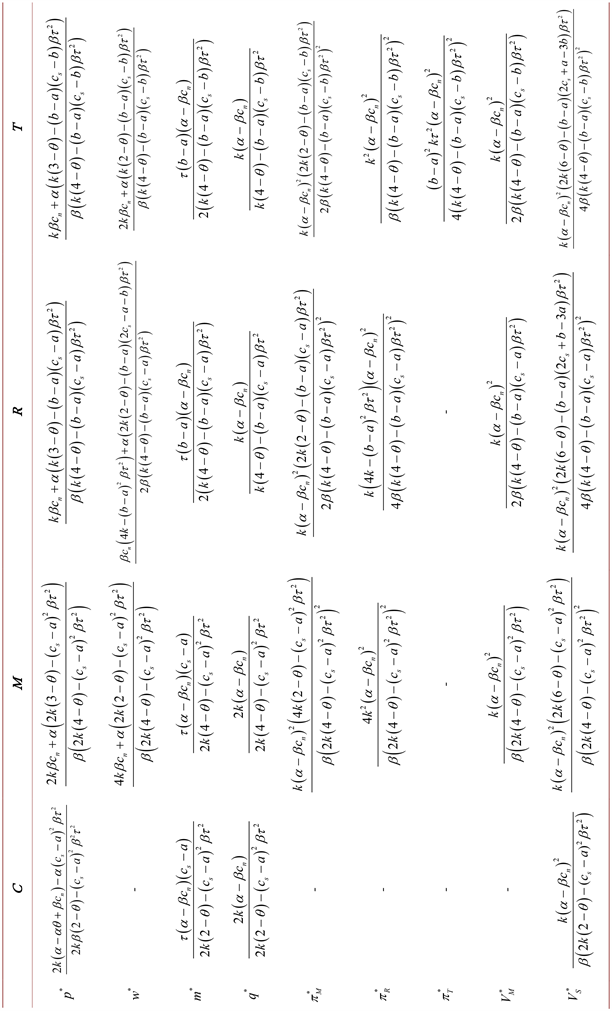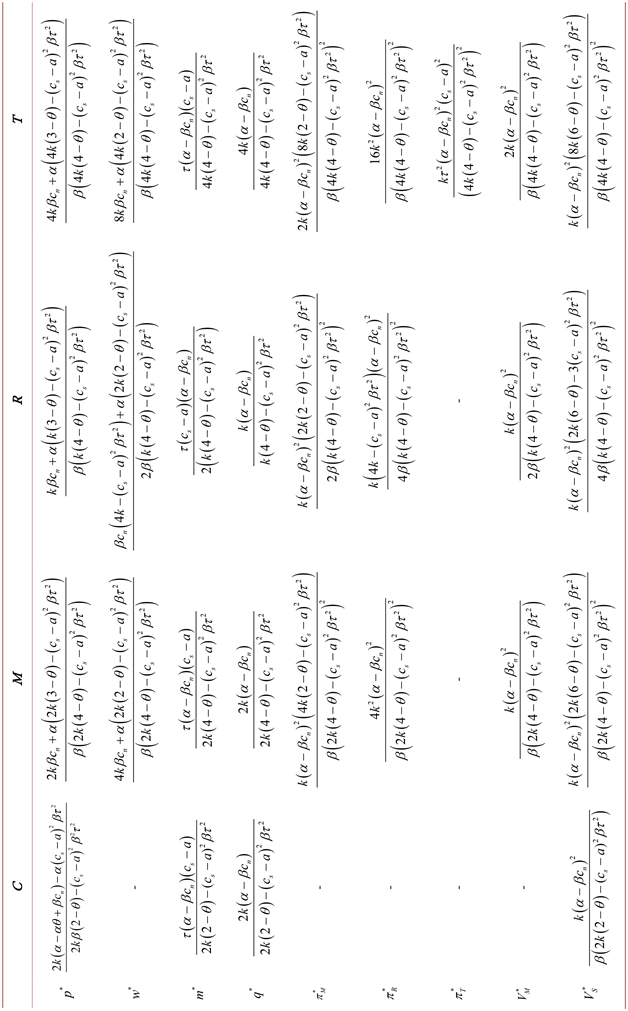$\frac{\partial {m}_{c}^{*}}{\partial \tau }>0$, $\frac{\partial {m}_{M}^{*}}{\partial \tau }>0$, $\frac{\partial {m}_{R}^{*}}{\partial \tau }>0$, $\frac{\partial {m}_{T}^{*}}{\partial \tau }>0$.

$\frac{\partial {m}_{c}^{*}}{\partial \theta }=\frac{2k\tau \left(\alpha -\beta {c}_{n}\right)\left({c}_{s}-a\right)}{{\left(2k\left(2-\theta \right)-\beta {\tau }^{2}{\left({c}_{s}-a\right)}^{2}\right)}^{2}}>0$,

$\frac{\partial {m}_{c}^{*}}{\partial \tau }=\frac{\left(\alpha -\beta {c}_{n}\right)\left({c}_{s}-a\right)\left(2k\left(2-\theta \right)+{\left({c}_{s}-a\right)}^{2}\beta {\tau }^{2}\right)}{{\left(2k\left(2-\theta \right)-\beta {\tau }^{2}{\left({c}_{s}-a\right)}^{2}\right)}^{2}}>0$,

$\frac{\partial {q}_{c}^{*}}{\partial \theta }>0$, $\frac{\partial {q}_{M}^{*}}{\partial \theta }>0$, $\frac{\partial {q}_{R}^{*}}{\partial \theta }>0$, $\frac{\partial {q}_{T}^{*}}{\partial \theta }>0$,

$\frac{\partial {p}_{c}^{*}}{\partial \theta }<0$, $\frac{\partial {p}_{M}^{*}}{\partial \theta }<0$, $\frac{\partial {p}_{R}^{*}}{\partial \theta }<0$, $\frac{\partial {p}_{T}^{*}}{\partial \theta }<0$.

$\frac{\partial {w}_{M}^{*}}{\partial \theta }=-\frac{8{k}^{2}\left(\alpha -\beta {c}_{n}\right)}{\beta {\left(2k\left(4-\theta \right)-{\left({c}_{s}-a\right)}^{2}\beta {\tau }^{2}\right)}^{2}}<0$

$\frac{\partial {q}_{c}^{*}}{\partial \theta }=\frac{4{k}^{2}\left(\alpha -\beta {c}_{n}\right)}{{\left(2k\left(2-\theta \right)-\beta {\tau }^{2}{\left({c}_{s}-a\right)}^{2}\right)}^{2}}>0$,

$\frac{\partial {p}_{c}^{*}}{\partial \theta }=-\frac{2k\left(2k\left(\alpha -\beta {c}_{n}\right)+\alpha \left(\beta -1\right){\tau }^{2}{\left({c}_{s}-a\right)}^{2}\right)}{\beta {\left(2k\left(2-\theta \right)-{\tau }^{2}{\left({c}_{s}-a\right)}^{2}\right)}^{2}}<0$,

$\frac{\partial {\pi }_{MR}^{*}}{\partial \theta }>0$, $\frac{\partial {\pi }_{RR}^{*}}{\partial \theta }>0$, $\frac{\partial {\pi }_{TR}^{*}}{\partial \theta }>0$, $\frac{\partial {\pi }_{TT}^{*}}{\partial \theta }>0$,

$\frac{\partial {V}_{MM}^{*}}{\partial \theta }>0$, $\frac{\partial {V}_{RM}^{*}}{\partial \theta }>0$, $\frac{\partial {V}_{TM}^{*}}{\partial \theta }>0$,

$\frac{\partial {V}_{CS}^{*}}{\partial \theta }>0$, $\frac{\partial {V}_{MS}^{*}}{\partial \theta }>0$, $\frac{\partial {V}_{RS}^{*}}{\partial \theta }>0$, $\frac{\partial {V}_{TS}^{*}}{\partial \theta }>0$.

$\frac{\partial {\pi }_{MM}^{*}}{\partial \theta }=-\frac{8{k}^{3}\theta {\left(\alpha -\beta {c}_{n}\right)}^{2}}{\beta {\left(2k\left(4-\theta \right)-\beta {\tau }^{2}{\left({c}_{s}-a\right)}^{2}\right)}^{3}}<0$,

$\frac{\partial {\pi }_{MR}^{*}}{\partial \theta }=\frac{16{k}^{3}{\left(\alpha -\beta {c}_{n}\right)}^{2}}{\beta {\left(2k\left(4-\theta \right)-\beta {\tau }^{2}{\left({c}_{s}-a\right)}^{2}\right)}^{3}}>0$,

$\frac{\partial {\pi }_{TT}^{*}}{\partial \theta }=\frac{8{k}^{2}{\tau }^{2}{\left(\alpha -\beta {c}_{n}\right)}^{2}{\left({c}_{s}-a\right)}^{2}}{{\left(4k\left(4-\theta \right)-\beta {\tau }^{2}{\left({c}_{s}-a\right)}^{2}\right)}^{3}}>0$

$\frac{\partial {V}_{MM}^{*}}{\partial \theta }=\frac{2{k}^{2}{\left(\alpha -\beta {c}_{n}\right)}^{2}}{\beta {\left(2k\left(4-\theta \right)-\beta {\tau }^{2}{\left(-a+{c}_{s}\right)}^{2}\right)}^{2}}>0$

$\frac{\partial {V}_{CS}^{*}}{\partial \theta }=\frac{4{k}^{2}{\left(\alpha -\beta {c}_{n}\right)}^{2}}{\beta {\left(2k\left(2-\theta \right)-\beta {\tau }^{2}{\left({c}_{s}-a\right)}^{2}\right)}^{3}}>0$

${m}_{c}^{*}>{m}_{R}^{*}>{m}_{M}^{*}>{m}_{T}^{*}$ ;

${q}_{c}^{*}>{q}_{R}^{*}>{q}_{M}^{*}>{q}_{T}^{*}$ ;

${p}_{c}^{*}<{p}_{R}^{*}<{p}_{M}^{*}<{p}_{T}^{*}$.

${w}_{T}^{*}-{w}_{M}^{*}=\frac{4k{\tau }^{2}\left(\alpha -\beta {c}_{n}\right){\left({c}_{s}-a\right)}^{2}}{\left(2k\left(4-\theta \right)-\beta {\tau }^{2}{\left({c}_{s}-a\right)}^{2}\right)\left(4k\left(4-\theta \right)-\beta {\tau }^{2}{\left({c}_{s}-a\right)}^{2}\right)}>0$,

${w}_{R}^{*}-{w}_{M}^{*}=\frac{{\tau }^{2}\left(\alpha -\beta {c}_{n}\right){\left({c}_{s}-a\right)}^{2}\left(2k\left(2-\theta \right)-\beta {\tau }^{2}{\left({c}_{s}-a\right)}^{2}\right)}{2\left(k\left(4-\theta \right)-\beta {\tau }^{2}{\left({c}_{s}-a\right)}^{2}\right)\left(2k\left(4-\theta \right)-\beta {\tau }^{2}{\left({c}_{s}-a\right)}^{2}\right)}>0$,

${w}_{T}^{*}-{w}_{R}^{*}=-\frac{{\tau }^{2}\left(\alpha -\beta {c}_{n}\right){\left({c}_{s}-a\right)}^{2}\left(4k\left(1-\theta \right)-\beta {\tau }^{2}{\left({c}_{s}-a\right)}^{2}\right)}{2\left(k\left(4-\theta \right)-\beta {\tau }^{2}{\left({c}_{s}-a\right)}^{2}\right)\left(4k\left(4-\theta \right)-\beta {\tau }^{2}{\left({c}_{s}-a\right)}^{2}\right)}={f}_{1}\left(\theta \right)$,

${f}_{1}\left(\theta \right)$$\theta \in \left(0,1\right)$ 单调递增。令 ${f}_{1}\left(\theta \right)=0$ 求得 ${\theta }^{*}=1-\frac{\beta {\tau }^{2}{\left({c}_{s}-a\right)}^{2}}{4k}$，所以当 $0<\theta <{\theta }^{*}$ 时，有 ${w}_{R}^{*}>{w}_{T}^{*}>{w}_{M}^{*}$ ；当 ${\theta }^{*}\le \theta <1$ 时，有 ${w}_{T}^{*}>{w}_{R}^{*}>{w}_{M}^{*}$，故定理1得证。

② 当 $k>\mathrm{max}\left\{\frac{\beta {\tau }^{2}{\left({c}_{s}-a\right)}^{2}+\tau \left(\alpha -\beta {c}_{n}\right)\left({c}_{s}-a\right)}{2\left(2-\theta \right)},\frac{3\beta {\tau }^{2}{\left({c}_{s}-a\right)}^{2}\left(2-\theta \right)}{\left(4-\theta \right)\left(4-3\theta \right)}\right\}$ 时，有 ${\pi }_{RM}^{*}>{\pi }_{MM}^{*}>{\pi }_{TM}^{*}$

${V}_{RM}^{*}>{V}_{MM}^{*}>{V}_{TM}^{*}$

${\pi }_{MR}^{*}-{\pi }_{TR}^{*}=\frac{4{k}^{2}{\tau }^{2}{\left(\alpha -\beta {c}_{n}\right)}^{2}{\left({c}_{s}-a\right)}^{2}\left(8k\left(4-\theta \right)-3\beta {\tau }^{2}{\left({c}_{s}-a\right)}^{2}\right)}{{\left(4k\left(4-\theta \right)-\beta {\tau }^{2}{\left({c}_{s}-a\right)}^{2}\right)}^{2}{\left(2k\left(4-\theta \right)-\beta {\tau }^{2}{\left({c}_{s}-a\right)}^{2}\right)}^{2}}>0$,

${\pi }_{RR}^{*}-{\pi }_{MR}^{*}=\frac{k{\tau }^{2}{\left(\alpha -\beta {c}_{n}\right)}^{2}{\left({c}_{s}-a\right)}^{2}\left(4{k}^{2}\left(4-\theta \right)\theta +4k\left(1-\theta \right)\beta {\tau }^{2}{\left({c}_{s}-a\right)}^{2}-{\beta }^{2}{\tau }^{4}{\left({c}_{s}-a\right)}^{4}\right)}{4{\left(k\left(4-\theta \right)-\beta {\tau }^{2}{\left({c}_{s}-a\right)}^{2}\right)}^{2}{\left(2k\left(4-\theta \right)-\beta {\tau }^{2}{\left({c}_{s}-a\right)}^{2}\right)}^{2}}>0$,

${\pi }_{MM}^{*}-{\pi }_{TM}^{*}=\frac{k{\tau }^{2}{\left(\alpha -\beta {c}_{n}\right)}^{2}{\left({c}_{s}-a\right)}^{2}\left(8{k}^{2}\left(4-\theta \right)\left(4-3\theta \right)-12k\left(2-\theta \right)\beta {\tau }^{2}{\left({c}_{s}-a\right)}^{2}+{\beta }^{2}{\tau }^{4}{\left({c}_{s}-a\right)}^{4}\right)}{{\left(4k\left(4-\theta \right)-\beta {\tau }^{2}{\left({c}_{s}-a\right)}^{2}\right)}^{2}{\left(2k\left(4-\theta \right)-\beta {\tau }^{2}{\left({c}_{s}-a\right)}^{2}\right)}^{2}}>0$

${\pi }_{RM}^{*}-{\pi }_{MM}^{*}=\frac{k{\tau }^{2}{\left(\alpha -\beta {c}_{n}\right)}^{2}{\left({c}_{s}-a\right)}^{2}\left(2k\left(k\left(4-\theta \right)\left(4-3\theta \right)-3\left(2-\theta \right)\beta {\tau }^{2}{\left({c}_{s}-a\right)}^{2}\right)+{\beta }^{2}{\tau }^{4}{\left({c}_{s}-a\right)}^{4}\right)}{{\left(2k\left(4-\theta \right)-\beta {\tau }^{2}{\left({c}_{s}-a\right)}^{2}\right)}^{2}2{\left(k\left(4-\theta \right)-\beta {\tau }^{2}{\left({c}_{s}-a\right)}^{2}\right)}^{2}}$

${V}_{MM}^{*}-{V}_{TM}^{*}=\frac{k{\tau }^{2}{\left(\alpha -\beta {c}_{n}\right)}^{2}{\left({c}_{s}-a\right)}^{2}}{\left(4k\left(4-\theta \right)-\beta {\tau }^{2}{\left({c}_{s}-a\right)}^{2}\right)\left(2k\left(4-\theta \right)-\beta {\tau }^{2}{\left({c}_{s}-a\right)}^{2}\right)}>0$${V}_{RM}^{*}-{V}_{MM}^{*}=\frac{k{\tau }^{2}{\left(\alpha -\beta {c}_{n}\right)}^{2}{\left({c}_{s}-a\right)}^{2}}{2\left(k\left(4-\theta \right)-\beta {\tau }^{2}{\left({c}_{s}-a\right)}^{2}\right)\left(2k\left(4-\theta \right)-\beta {\tau }^{2}{\left({c}_{s}-a\right)}^{2}\right)}>0$，故有 ${V}_{RM}^{*}>{V}_{MM}^{*}>{V}_{TM}^{*}$

${V}_{MS}^{*}-{V}_{TS}^{*}=\frac{2{k}^{2}{\tau }^{2}{\left(\alpha -\beta {c}_{n}\right)}^{2}{\left({c}_{s}-a\right)}^{2}\left(2k\left(12-\theta \right)\left(4-\theta \right)-\beta {\tau }^{2}\left(10-\theta \right){\left({c}_{s}-a\right)}^{2}\right)}{{\left(2k\left(4-\theta \right)-\beta {\tau }^{2}{\left({c}_{s}-a\right)}^{2}\right)}^{2}{\left(4k\left(4-\theta \right)-\beta {\tau }^{2}{\left({c}_{s}-a\right)}^{2}\right)}^{2}}>0$，即 ${V}_{MS}^{*}>{V}_{TS}^{*}$

${V}_{RS}^{*}-{V}_{MS}^{*}=\frac{k{\tau }^{2}{\left(\alpha -\beta {c}_{n}\right)}^{2}{\left({c}_{s}-a\right)}^{2}\left(2k\left(8k\left(4-\theta \right)-\beta {\tau }^{2}{\left({c}_{s}-a\right)}^{2}\left(10-\theta \right)\right)+{\beta }^{2}{\tau }^{4}{\left({c}_{s}-a\right)}^{4}\right)}{4{\left(k\left(4-\theta \right)-\beta {\tau }^{2}{\left({c}_{s}-a\right)}^{2}\right)}^{2}{\left(2k\left(4-\theta \right)-\beta {\tau }^{2}{\left({c}_{s}-a\right)}^{2}\right)}^{2}}$

② 在制造商或零售商负责回收时，当 $0<\theta <{\theta }^{**}$ 时，有 ${\pi }_{MM}^{*}>{\pi }_{MR}^{*}$ ( ${\pi }_{RM}^{*}>{\pi }_{RR}^{*}$ )；当 ${\theta }^{**}\le \theta <1$ 时，有 ${\pi }_{MR}^{*}>{\pi }_{MM}^{*}$，( ${\pi }_{RR}^{*}>{\pi }_{RM}^{*}$ )，其中 ${\theta }^{**}=1-\frac{\beta {\tau }^{2}{\left({c}_{s}-a\right)}^{2}}{4k}$ ；在第三方负责回收时，当 $0<\theta <{\theta }^{***}$ 时，有 ${\pi }_{TM}^{*}>{\pi }_{TR}^{*}>{\pi }_{TT}^{*}$ ；当 ${\theta }^{***}\le \theta <1$ 时，有 ${\pi }_{TR}^{*}>{\pi }_{TM}^{*}>{\pi }_{TT}^{*}$，其中 ${\theta }^{***}=1-\frac{\beta {\tau }^{2}{\left({c}_{s}-a\right)}^{2}}{8k}$

${V}_{TM}^{*}-{\pi }_{TR}^{*}=\frac{2k{\left(\alpha -\beta {c}_{n}\right)}^{2}\left(4k\left(2-\theta \right)-\beta {\tau }^{2}{\left({c}_{s}-a\right)}^{2}\right)}{\beta {\left(4k\left(4-\theta \right)-\beta {\tau }^{2}{\left({c}_{s}-a\right)}^{2}\right)}^{2}}>0$,

${V}_{TM}^{*}-{\pi }_{TT}^{*}=\frac{k{\left(\alpha -\beta {c}_{n}\right)}^{2}\left(8k\left(4-\theta \right)-3\beta {\tau }^{2}{\left({c}_{s}-a\right)}^{2}\right)}{\beta {\left(4k\left(4-\theta \right)-\beta {\tau }^{2}{\left({c}_{s}-a\right)}^{2}\right)}^{2}}>0$,

② 根据表2的均衡结果，证明过程如下：

${\pi }_{MR}^{*}-{\pi }_{MM}^{*}=-\frac{k{\left(\alpha -\beta {c}_{n}\right)}^{2}\left(4k\left(1-\theta \right)-\beta {\tau }^{2}{\left({c}_{s}-a\right)}^{2}\right)}{\beta {\left(2k\left(4-\theta \right)-\beta {\tau }^{2}{\left({c}_{s}-a\right)}^{2}\right)}^{2}}={f}_{2}\left(\theta \right)$,

${f}_{2}\left(\theta \right)$ 进行求导可得 ${{f}^{\prime }}_{2}\left(\theta \right)=\frac{8{k}^{3}\left(2+\theta \right){\left(\alpha -\beta {c}_{n}\right)}^{2}}{\beta {\left(2k\left(4-\theta \right)-\beta {\tau }^{2}{\left({c}_{s}-a\right)}^{2}\right)}^{3}}>0$，因此 ${f}_{2}\left(\theta \right)$$\theta \in \left(0,1\right)$ 单调递增，故令 ${f}_{2}\left(\theta \right)=0$ 可求得 ${\theta }^{**}=1-\frac{\beta {\tau }^{2}{\left({c}_{s}-a\right)}^{2}}{4k}$。所以当 $0<\theta <{\theta }^{**}$ 时，有 ${\pi }_{MM}^{*}>{\pi }_{MR}^{*}$ ；当 ${\theta }^{**}\le \theta <1$ 时，有 ${\pi }_{MR}^{*}>{\pi }_{MM}^{*}$

${\pi }_{TR}^{*}-{\pi }_{TT}^{*}=\frac{k{\left(\alpha -\beta {c}_{n}\right)}^{2}\left(16k-\beta {\tau }^{2}{\left({c}_{s}-a\right)}^{2}\right)}{\beta {\left(4k\left(4-\theta \right)-\beta {\tau }^{2}{\left({c}_{s}-a\right)}^{2}\right)}^{2}}>0$,

${\pi }_{TM}^{*}-{\pi }_{TT}^{*}=\frac{k{\left(\alpha -\beta {c}_{n}\right)}^{2}\left(16k\left(2-\theta \right)-3\beta {\tau }^{2}{\left({c}_{s}-a\right)}^{2}\right)}{\beta {\left(4k\left(4-\theta \right)-\beta {\tau }^{2}{\left({c}_{s}-a\right)}^{2}\right)}^{2}}>0$,

${\pi }_{TR}^{*}-{\pi }_{TM}^{*}=\frac{2k{\left(\alpha -\beta {c}_{n}\right)}^{2}\left(8k\left(\theta -1\right)+\beta {\tau }^{2}{\left({c}_{s}-a\right)}^{2}\right)}{\beta {\left(4k\left(4-\theta \right)-\beta {\tau }^{2}{\left({c}_{s}-a\right)}^{2}\right)}^{2}}={f}_{3}\left(\theta \right)$,

${f}_{3}\left(\theta \right)$ 进行求导可得 ${{f}^{\prime }}_{3}\left(\theta \right)=\frac{64{k}^{3}\left(2+\theta \right){\left(\alpha -\beta {c}_{n}\right)}^{2}}{\beta {\left(4k\left(4-\theta \right)-\beta {\tau }^{2}{\left(a-{c}_{s}\right)}^{2}\right)}^{3}}>0$，因此 ${f}_{3}\left(\theta \right)$$\theta \in \left(0,1\right)$ 单调递增，故令 ${f}_{3}\left(\theta \right)=0$ 可求得 ${\theta }^{***}=1-\frac{\beta {\tau }^{2}{\left({c}_{s}-a\right)}^{2}}{8k}$。所以当 $0<\theta <{\theta }^{***}$ 时，有 ${\pi }_{TM}^{*}>{\pi }_{TR}^{*}>{\pi }_{TT}^{*}$ ；当 ${\theta }^{***}\le \theta <1$ 时，有 ${\pi }_{TR}^{*}>{\pi }_{TM}^{*}>{\pi }_{TT}^{*}$

5. 数值仿真及实证分析

5.1. 数值仿真分析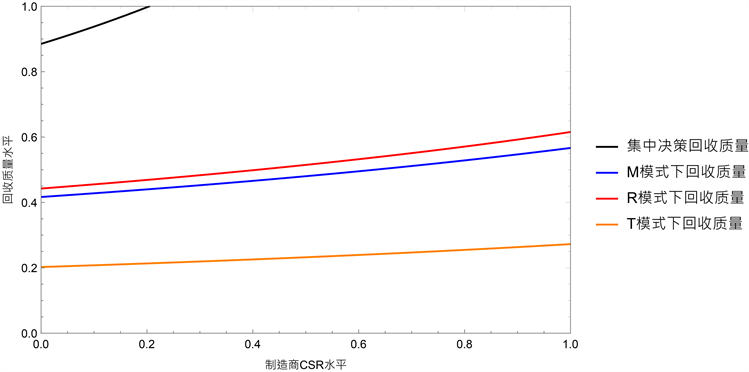Figure 1. Recovery quality level of three recovery modes under different CSR levels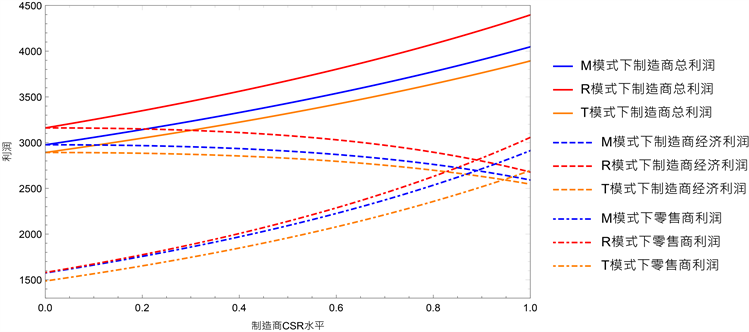Figure 2. Profit comparison of three recovery models under different CSR levels

5.2. 实证分析Table 3. Comparison of the best recycling quality and retail price of waste products under three recycling modes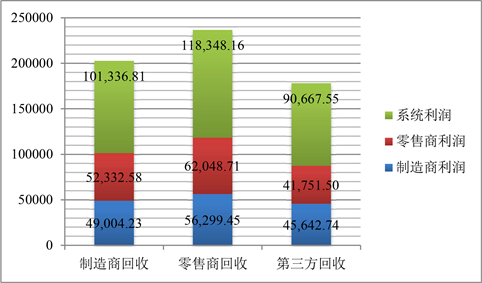Figure 3. Profit comparison of closed-loop supply chain entities under different recovery modes

6. 结论

1) 无论在哪种回收模式下，制造商进行CSR的投入均有利于废旧产品回收质量的提升，尽管此时制造商的成本优势会转移至回收方，但是其对于正向供应链中市场需求的影响是正向的；且回收率的提升在一定程度上也能够提升废旧产品的回收质量；

2) 无论在哪种回收模式下，随着制造商CSR投入程度的不断增强，为挽回一定经济利润损失的制造商会降低产品的批发价格，由此零售商决定的产品的零售价格会逐渐下降，从而有助于扩大市场需求；

3) 无论在哪种回收模式下，随着制造商CSR投入程度的不断增强，在正向供应链中由于市场需求的提升制造商的总利润、零售商的利润及系统的总利润均会有显著提升，但是会损害制造商自身的经济利润；

4) 无论是从降低零售价格能够更好地刺激市场需求角度，还是从提升废旧产品的回收质量使得制造商及零售商经济利润有所提升的角度，当制造商进行CSR投入时零售商回收模式是最优的，第三方回收模式效果是最差的。

  Friedman, M. (2007) The Social Responsibility of Business Is to Increase Its Profits. In: Corporate Ethics and Corporate Governance, Springer, Berlin, 173-178. https://doi.org/10.1007/978-3-540-70818-6_14  Becker-Olsen, K.L., Cudmore, B.A. and Hill, R.P. (2006) The Impact of Perceived Corporate Social Responsibility on Consumer Behavior. Journal of Business Research, 59, 46-53. https://doi.org/10.1016/j.jbusres.2005.01.001  Savaskan, R.C. and Van Wassenhove, L.N. (2006) Reverse Channel Design: The Case of Competing Retailers. Management Science, 52, 1-14. https://doi.org/10.1287/mnsc.1050.0454  Atasu, A., Toktay, L.B. and Van Wassenhove, L.N. (2013) How Collection Cost Structure Drives a Manufacturer’s Reverse Channel Choice. Production and Operations Management, 22, 1089-1102. https://doi.org/10.1111/j.1937-5956.2012.01426.x  周雄伟, 熊花纬, 陈晓红. 基于回收产品质量水平的闭环供应链渠道选择模型[J]. 控制与决策, 2017, 32(2): 193-202.  Xing, K., Belusko, M., Luong, L., et al. (2007) An Evaluation Model of Product Upgradeability for Remanufacture. The International Journal of Advanced Manufacturing Technology, 35, 1-14. https://doi.org/10.1007/s00170-006-0698-9  李舒玲. 制造商绿色偏好下的废旧产品质量供应链决策[J]. 物流技术, 2018, 37(5): 101-106, 123.  邹清明, 陈建华, 叶广宇. 考虑产品设计和回收质量的闭环供应链的定价与协调[J]. 系统工程, 2017, 35(5): 140-144.  刘家国, 周学龙, 赵金楼. 基于产品质量差异的闭环供应链定价策略与协调研究[J]. 中国管理科学, 2013, 21(S2): 426-431.  Bayındır, Z.P., Erkip, N. and Güllü, R. (2007) Assessing the Benefits of Remanufacturing Option under One-Way Substitution and Capacity Constraint. Computers & Operations Research, 34, 487-514. https://doi.org/10.1016/j.cor.2005.03.010  Chen, W., Kucukyazici, B. and Verter, V. (2015) Supply Chain Design for Unlocking the Value of Remanufacturing Underuncertainty. European Journal of Operational Research, 247, 804-819. https://doi.org/10.1016/j.ejor.2015.06.062  Liu, L.W., Wang, Z.J., Xu, L., et al. (2017) Collection Effort and Reverse Channel Choices in a Closed-Loop Supply Chain. The Journal of Cleaner Production, 144, 492-500. https://doi.org/10.1016/j.jclepro.2016.12.126  Amaeshi, K.M., Osuji, O.K. and Nnodim, P. (2008) Corporate Social Responsibility in Supply Chains of Global Brands: A Boundaryless Responsibility? Clarifications, Exceptions and Implications. Journal of Business Ethics, 81, 23-234. https://doi.org/10.1007/s10551-007-9490-5  Modak, N.M., Kazemi, N. and Cárdenas Barrónc, L.E. (2019) Investigating Structure of a Two-Echelon Closed-Loop Supply Chain Using Social Work Donation as a Corporate Social Responsibility Practice. International Journal of Production Economics, 207, 19-33. https://doi.org/10.1016/j.ijpe.2018.10.009  郑本荣, 杨超, 杨珺. CSR投入对闭环供应链定价与协调决策的影响[J]. 中国管理科学, 2018, 26(10): 64-78.  姚锋敏, 王悦, 滕春贤. 考虑企业社会责任的闭环供应链销售努力与定价决策[J]. 计算机集成制造系统, 2020, 26(3): 839-848.  Song, L., Yan, Y. and Yao, F. (2020) Closed-Loop Supply Chain Models Considering Government Subsidy and Corporate Social Responsibility Investment. Sustainability, 12, 2045. https://doi.org/10.3390/su12052045  姚锋敏, 刘珊, 陈东彦, 等. 具有企业社会责任的闭环供应链回收及定价决策[J]. 控制与决策, 2019, 34(9): 1981-1990.